• Slides: 13Graphing a Quadratic Equation Review • The anatomy of a parabola: Zeros, Roots, Solution, x-intercepts Vertex y-intercept Axis of Symmetry Increasing Interval Decreasing Interval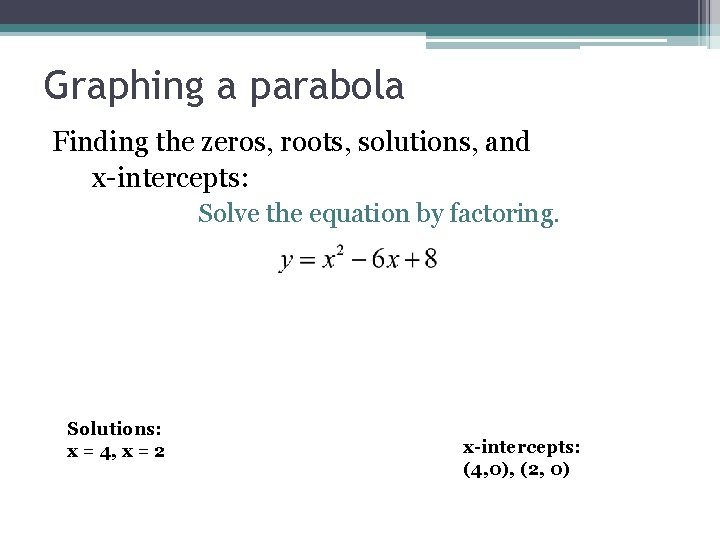Graphing a parabola Finding the zeros, roots, solutions, and x-intercepts: Solve the equation by factoring. Solutions: x = 4, x = 2 x-intercepts: (4, 0), (2, 0)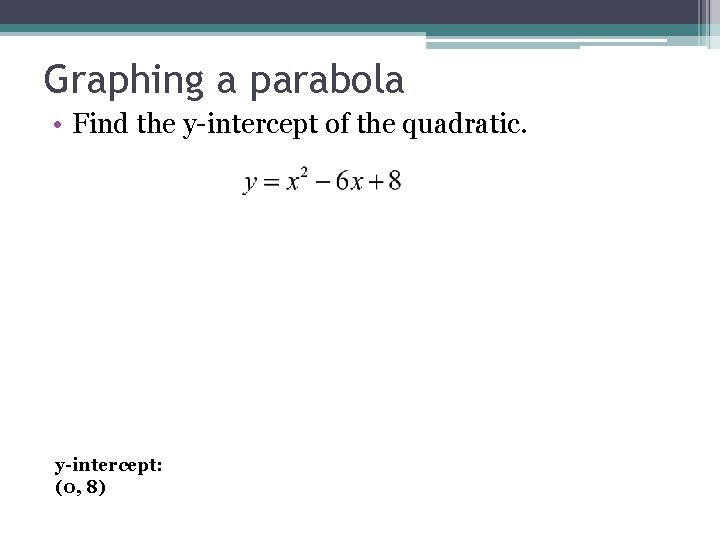Graphing a parabola • Find the y-intercept of the quadratic. y-intercept: (0, 8)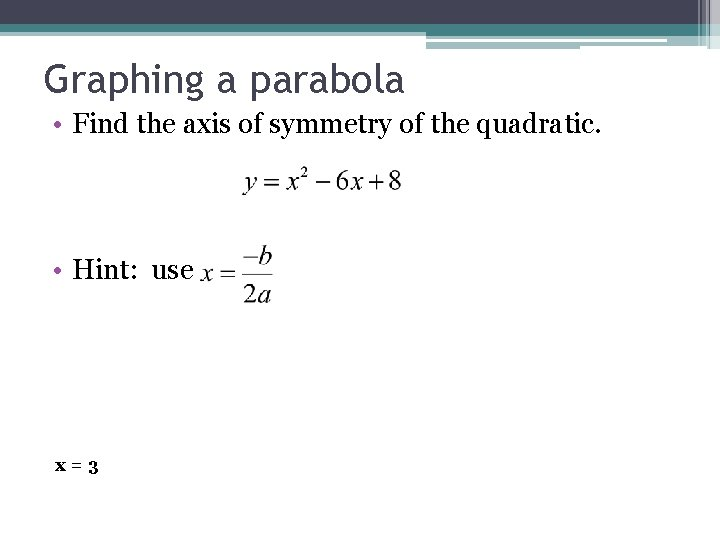Graphing a parabola • Find the axis of symmetry of the quadratic. • Hint: use x=3Graphing a parabola • Solving for the vertex. Vertex is (3, -1)Graphing a parabola • Determine if the parabola has a maximum or a minimum and the value. Minimum, -1Put it all together to graph Increasing Interval: Decreasing Interval: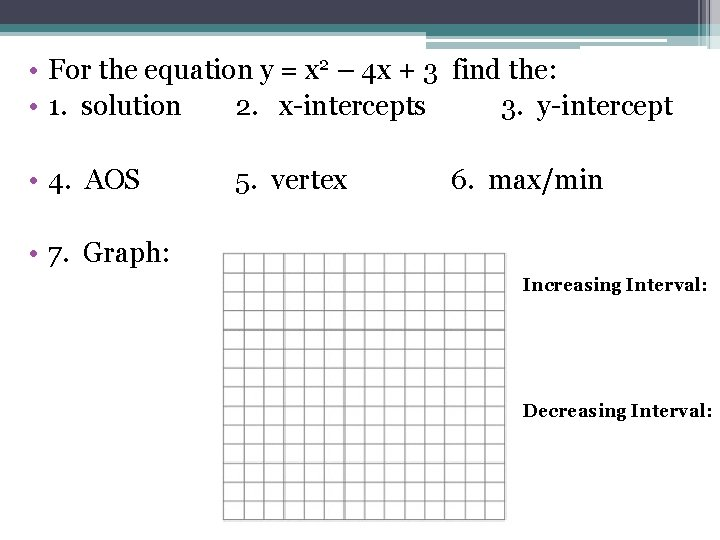• For the equation y = x 2 – 4 x + 3 find the: • 1. solution 2. x-intercepts 3. y-intercept • 4. AOS 5. vertex 6. max/min • 7. Graph: Increasing Interval: Decreasing Interval: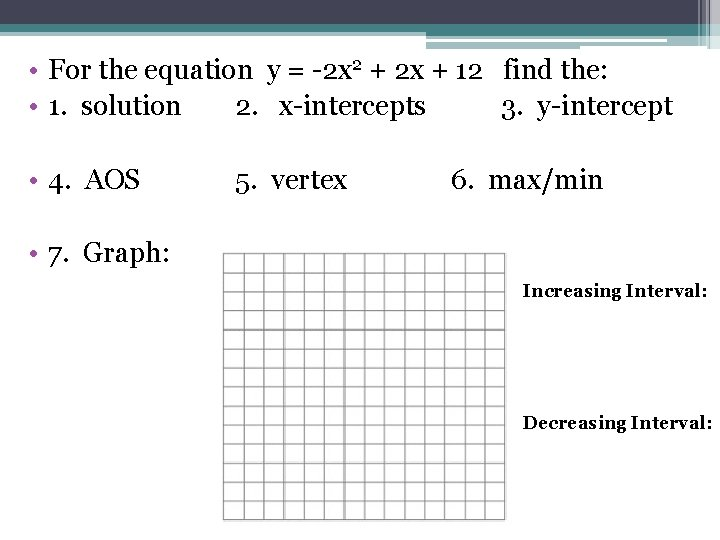• For the equation y = -2 x 2 + 2 x + 12 find the: • 1. solution 2. x-intercepts 3. y-intercept • 4. AOS 5. vertex 6. max/min • 7. Graph: Increasing Interval: Decreasing Interval:• For the equation y = 15 x 2 + x find the: • 1. solution 2. x-intercepts 3. y-intercept • 4. AOS 5. vertex 6. max/min • 7. Graph: Increasing Interval: Decreasing Interval: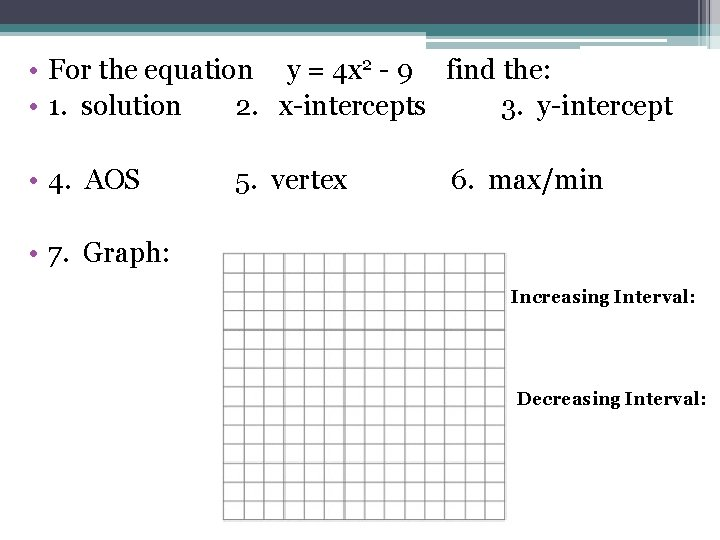• For the equation y = 4 x 2 - 9 find the: • 1. solution 2. x-intercepts 3. y-intercept • 4. AOS 5. vertex 6. max/min • 7. Graph: Increasing Interval: Decreasing Interval:• For the equation y = 2 x 2 + 3 x + 1 find the: • 1. solution 2. x-intercepts 3. y-intercept • 4. AOS 5. vertex 6. max/min • 7. Graph: Increasing Interval: Decreasing Interval: